# Numbers

### In Norwegian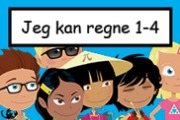### Jeg kan regne

Learn the numbers from 1 to 10. Addition and subtraction.### Jeg kan regne 1-4### Matematikk.org

Mastering Arithmetic – Year 1### Matematikk.org

Mastering Arithmetic – Year 2### Matematikk.org

Mastering Arithmetic – Years 3–4### NRK Super

Mattekode-x is a mathematics programme for 6–7-year-olds, designed specifically for children starting school.### NRK Super

Tellekorpset (TV show) Fun with numbers and counting, amounts and sizes.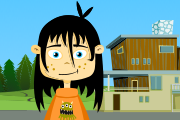### Salaby

Mathematics 1–2 Various exercises and games.

### In English and Norwegian### Kikora

Mathematic assignments about numbers, the four basic arithmetic and more.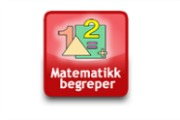### Tema morsmål

Illustrated list of mathematical terms in English and Norwegian (bokmål).### Tema morsmål

Illustrated list of mathematical terms in English and Norwegian (nynorsk).

### In Norwegian### Kikora

Mathematic assignments about numbers, the four basic arithmetic and more.### Matematikk.org

Mastering Arithmetic – Years 5–6### Matematikk.org

Mastering Arithmetic – Year 7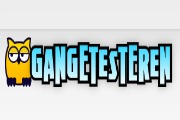### Matematikk.org

Master the small and large times tables.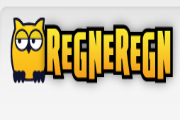### Matematikk.org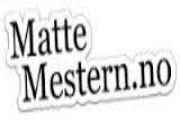### MatteMestern

Learn mathematics by watching, listening and doing exercises in Norwegian.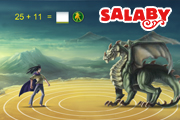### Salaby

Various maths exercises.### Tema morsmål

Illustrated list of mathematical terms in English and Norwegian (bokmål).### Tema morsmål

Illustrated list of mathematical terms in English and Norwegian (nynorsk).

### In English and Norwegian### Kikora

Matteoppgaver innen temaene titallsystem, romertall, primtall og mer.### Tema morsmål

Illustrated list of mathematical terms in English and Norwegian (bokmål).### Tema morsmål

Illustrated list of mathematical terms in English and Norwegian (nynorsk).

### In Norwegian### Brøk

Watch videos about fractions. From the project Fleksibel opplæring.### Desimaltall

Watch videos about decimal numbers. From the project Fleksibel opplæring.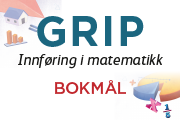### GRIP

Book with texts and exercises in counting, addition, subtraction, multiplication, division, positive and negative numbers, fractions, percentages, equations.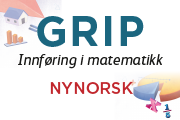### GRIP

Book with texts and exercises in counting, addition, subtraction, multiplication, division, positive and negative numbers, fractions, percentages, equations.### Heltall

Watch videos about whole numbers. From the project Fleksibel opplæring.### Kikora

Matteoppgaver innen temaene titallsystem, romertall, primtall og mer.### MatteMestern

Learn mathematics by watching, listening and doing exercises in Norwegian.### Prosent

Watch videos about percentages. From the project Fleksibel opplæring.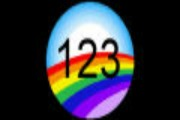### Sandefjordskolen

Practise the decimal system by choosing numbers: 1, 10, 100, 1000 …### Tema morsmål

Illustrated list of mathematical terms in English and Norwegian (bokmål).### Tema morsmål

Illustrated list of mathematical terms in English and Norwegian (nynorsk).